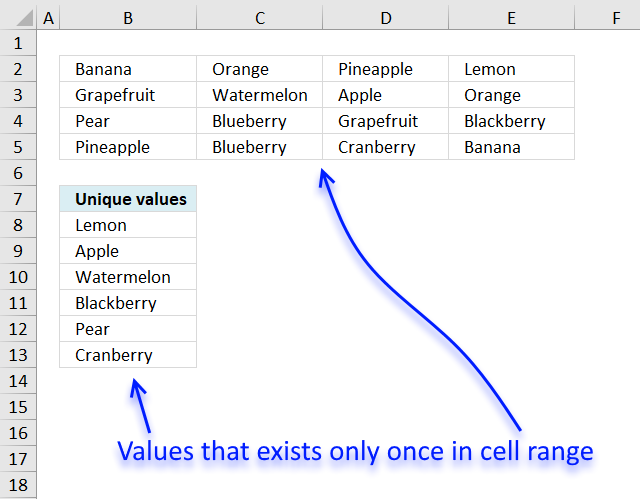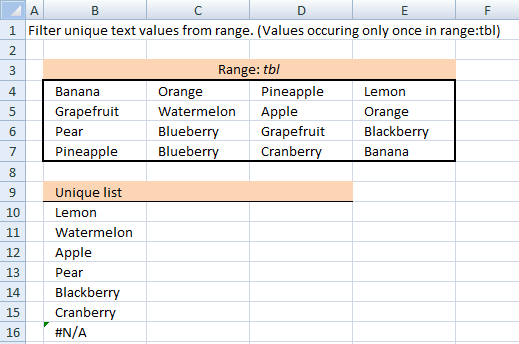Author: Oscar Cronquist Article last updated on February 09, 2019Unique values are values occurring only once in cell range. This is what I am going to demonstrate in this blog post using an array formula.

If you are looking for filtering unique distinct values sorted from A to Z, see this blog post: Extract a unique distinct list sorted from A-Z from range in excel

Array formula in cell B8:

=TEXTJOIN("", TRUE, IF(MIN(IF(((COUNTIF(\$B\$2:\$E\$5, \$B\$2:\$E\$5)=1)+COUNTIF(B7:\$B\$7, \$B\$2:\$E\$5))=1, (ROW(\$B\$2:\$E\$5)+(1/(COLUMN(\$B\$2:\$E\$5)+1)))*1, ""))=(ROW(\$B\$2:\$E\$5)+(1/(COLUMN(\$B\$2:\$E\$5)+1)))*1, \$B\$2:\$E\$5, ""))

To enter an array formula, type the formula in a cell then press and hold CTRL + SHIFT simultaneously, now press Enter once. Release all keys.

The formula bar now shows the formula with a beginning and ending curly bracket telling you that you entered the formula successfully. Don't enter the curly brackets yourself.

Explaining formula in cell B8

Step 1 - Identify unique values

The COUNTIF function counts values based on a condition or criteria, if number is 1 then value must be unique.

COUNTIF(\$B\$2:\$E\$5, \$B\$2:\$E\$5)=1

becomes

COUNTIF({"Banana", "Orange", "Pineapple", "Lemon";"Grapefruit", "Watermelon", "Apple", "Orange";"Pear", "Blueberry", "Grapefruit", "Blackberry";"Pineapple", "Blueberry", "Cranberry", "Banana"}, {"Banana", "Orange", "Pineapple", "Lemon";"Grapefruit", "Watermelon", "Apple", "Orange";"Pear", "Blueberry", "Grapefruit", "Blackberry";"Pineapple", "Blueberry", "Cranberry", "Banana"})=1

becomes

{2,2,2,1;2,1,1,2;1,2,2,1;2,2,1,2}=1

and returns

{FALSE, FALSE, FALSE, TRUE;FALSE, TRUE, TRUE, FALSE;TRUE, FALSE, FALSE, TRUE;FALSE, FALSE, TRUE, FALSE}.

Step 2 - Keep track of previous values

The first argument in the COUNTIF function contains an expanding cell reference, it grows when the cell is copied to cells below. This makes the formula aware of values displayed in cells above.

COUNTIF(B7:\$B\$7, \$B\$2:\$E\$5)

becomes

COUNTIF("Unique values", {"Banana", "Orange", "Pineapple", "Lemon";"Grapefruit", "Watermelon", "Apple", "Orange";"Pear", "Blueberry", "Grapefruit", "Blackberry";"Pineapple", "Blueberry", "Cranberry", "Banana"})

and returns

{0,0,0,0;0,0,0,0;0,0,0,0;0,0,0,0}.

First we add the arrays and then we check if a number is equal to 1. We then know that the value has not been shown and that it must be unique.

(COUNTIF(\$B\$2:\$E\$5, \$B\$2:\$E\$5)=1)+COUNTIF(B7:\$B\$7, \$B\$2:\$E\$5))=1

becomes

({FALSE, FALSE, FALSE, TRUE;FALSE, TRUE, TRUE, FALSE;TRUE, FALSE, FALSE, TRUE;FALSE, FALSE, TRUE, FALSE}+{0,0,0,0;0,0,0,0;0,0,0,0;0,0,0,0})=1

becomes

{0,0,0,1;0,1,1,0;1,0,0,1;0,0,1,0}=1

and returns

{FALSE, FALSE, FALSE, TRUE;FALSE, TRUE, TRUE, FALSE;TRUE, FALSE, FALSE, TRUE;FALSE, FALSE, TRUE, FALSE}.

Step 4 - Replace TRUE with unique number

The IF function returns unique number if boolean value is TRUE. FALSE returns "" (nothing).

IF(((COUNTIF(\$B\$2:\$E\$5, \$B\$2:\$E\$5)=1)+COUNTIF(B7:\$B\$7, \$B\$2:\$E\$5))=1, (ROW(\$B\$2:\$E\$5)+(1/(COLUMN(\$B\$2:\$E\$5)+1)))*1, "")

becomes

IF({FALSE, FALSE, FALSE, TRUE;FALSE, TRUE, TRUE, FALSE;TRUE, FALSE, FALSE, TRUE;FALSE, FALSE, TRUE, FALSE}, (ROW(\$B\$2:\$E\$5)+(1/(COLUMN(\$B\$2:\$E\$5)+1)))*1, "")

This part of the formula: (ROW(\$B\$2:\$E\$5)+(1/(COLUMN(\$B\$2:\$E\$5)+1)))*1 creates a unique value for each cell in cell range. This makes it easier to extract the correct value in a later step.

IF({FALSE, FALSE, FALSE, TRUE;FALSE, TRUE, TRUE, FALSE;TRUE, FALSE, FALSE, TRUE;FALSE, FALSE, TRUE, FALSE}, (ROW(\$B\$2:\$E\$5)+(1/(COLUMN(\$B\$2:\$E\$5)+1)))*1, "")

becomes

IF({FALSE, FALSE, FALSE, TRUE;FALSE, TRUE, TRUE, FALSE;TRUE, FALSE, FALSE, TRUE;FALSE, FALSE, TRUE, FALSE}, {2.33333333333333, 2.25, 2.2, 2.16666666666667;3.33333333333333, 3.25, 3.2, 3.16666666666667;4.33333333333333, 4.25, 4.2, 4.16666666666667;5.33333333333333, 5.25, 5.2, 5.16666666666667}, "")

and returns

{"","","",2.16666666666667;"",3.25,3.2,"";4.33333333333333,"","",4.16666666666667;"","",5.2,""}

Step 5 - Find smallest value in array

The MIN function returns the smallest number in array ignoring blanks and text values.

MIN(IF(((COUNTIF(\$B\$2:\$E\$5, \$B\$2:\$E\$5)=1)+COUNTIF(B7:\$B\$7, \$B\$2:\$E\$5))=1, (ROW(\$B\$2:\$E\$5)+(1/(COLUMN(\$B\$2:\$E\$5)+1)))*1, ""))

becomes

MIN({"","","",2.16666666666667;"",3.25,3.2,"";4.33333333333333,"","",4.16666666666667;"","",5.2,""})

and returns 2.16666666666667.

Step 4 - Find corresponding value

IF(MIN(IF(((COUNTIF(\$B\$2:\$E\$5, \$B\$2:\$E\$5)=1)+COUNTIF(B7:\$B\$7, \$B\$2:\$E\$5))=1, (ROW(\$B\$2:\$E\$5)+(1/(COLUMN(\$B\$2:\$E\$5)+1)))*1, ""))=(ROW(\$B\$2:\$E\$5)+(1/(COLUMN(\$B\$2:\$E\$5)+1)))*1, \$B\$2:\$E\$5, "")

becomes

IF(2.16666666666667=(ROW(\$B\$2:\$E\$5)+(1/(COLUMN(\$B\$2:\$E\$5)+1)))*1, \$B\$2:\$E\$5, "")

becomes

IF(2.16666666666667={2.33333333333333, 2.25, 2.2, 2.16666666666667;3.33333333333333, 3.25, 3.2, 3.16666666666667;4.33333333333333, 4.25, 4.2, 4.16666666666667;5.33333333333333, 5.25, 5.2, 5.16666666666667}, \$B\$2:\$E\$5, "")

becomes

IF({FALSE, FALSE, FALSE, TRUE;FALSE, FALSE, FALSE, FALSE;FALSE, FALSE, FALSE, FALSE;FALSE, FALSE, FALSE, FALSE}, \$B\$2:\$E\$5, "")

and returns

{"","","","Lemon";"","","","";"","","","";"","","",""}.

Step 5 - Concatenate strings in array

The TEXTJOIN function returns values concatenated ignoring blanks in array.

TEXTJOIN("", TRUE, IF(MIN(IF(((COUNTIF(\$B\$2:\$E\$5, \$B\$2:\$E\$5)=1)+COUNTIF(B7:\$B\$7, \$B\$2:\$E\$5))=1, (ROW(\$B\$2:\$E\$5)+(1/(COLUMN(\$B\$2:\$E\$5)+1)))*1, ""))=(ROW(\$B\$2:\$E\$5)+(1/(COLUMN(\$B\$2:\$E\$5)+1)))*1, \$B\$2:\$E\$5, ""))

becomes

TEXTJOIN("", TRUE, {"","","","Lemon";"","","","";"","","","";"","","",""})

and returns "Lemon" in cell B8.

Filter unique values from a range (for older Excel versions)Array formula in B10:

=INDEX(tbl, MIN(IF((COUNTIF(tbl, tbl)=1)*(COUNTIF(B\$9:\$B9, tbl)<>1), ROW(tbl)-MIN(ROW(tbl))+1)), MATCH(FALSE, COUNTIF(\$B\$9:B9, INDEX(tbl, MIN(IF((COUNTIF(tbl, tbl)=1)*(COUNTIF(B\$9:\$B9, tbl)<>1), ROW(tbl)-MIN(ROW(tbl))+1)), , 1))+COUNTIF(tbl, INDEX(tbl, MIN(IF((COUNTIF(tbl, tbl)=1)*(COUNTIF(B\$9:\$B9, tbl)<>1), ROW(tbl)-MIN(ROW(tbl))+1)), , 1))<>1, 0), 1) + CTRL + SHIFT + ENTER

copied down as far as necessary.

Named ranges

tbl (B4:E7)
What is named ranges?

How to implement array formula to your workbook

Change the named range. If your list starts at, for example, F3. Change \$B\$9:B9 in the above formulas to F2:\$F\$2.